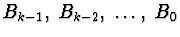CSE 5311 Fall 1999

Quiz #7

1.
(10 points). Prove the following property of binomial trees: for binomial tree Bk, the root has degree k, and the children of the root from left to right are binomial trees.

2.
(10 points). Show the Fibonacci Heap that results from each of the following operations: Insert(7), Insert(8), Insert(3), Insert(2), Insert(15), ExtractMin, Insert(10), Delete(15), ExtractMin